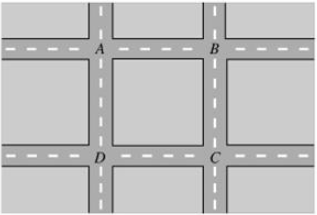# Problem: A man out walking his dog makes one complete pass around a perfectly square city block. He starts at point A and walks clockwise around the block. (Figure Below)Let  be the displacement vector from A to B,  be the displacement vector from B to C, etc.Part AWhich of the following vectors is equal to ?Which of the following vectors is equal to ?a)  onlyb)  onlyc)  onlyd) All of the abovee) None of the abovePart BWhich of the following vectors is equal to ?Which of the following vectors is equal to ?a)  onlyb)  onlyc)  onlyd) All of the abovee) None of the abovePart CWhich of the following vectors is equal to ?Which of the following vectors is equal to ?a)  onlyb)  onlyc)  onlyd) All of the abovee) None of the above

###### FREE Expert Solution

The vectors are represented as: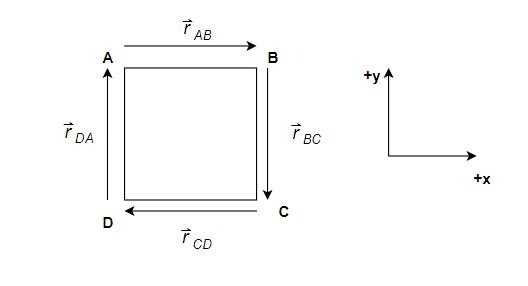91% (128 ratings)###### Problem Details

A man out walking his dog makes one complete pass around a perfectly square city block. He starts at point A and walks clockwise around the block. (Figure Below)

Let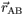be the displacement vector from A to B,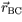be the displacement vector from B to C, etc.

Part A

Which of the following vectors is equal to?

Which of the following vectors is equal to ?

a)only

b)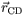only

c)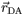only

d) All of the above

e) None of the above

Part B

Which of the following vectors is equal to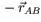?

Which of the following vectors is equal to ?

a)only

b)only

c)only

d) All of the above

e) None of the above

Part C

Which of the following vectors is equal to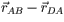?

Which of the following vectors is equal to ?

a)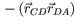only

b)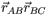only

c)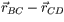only

d) All of the above

e) None of the above